C语言操作符详解（和bug郭一起学C系列）

• 时间:
• 浏览:• 操作符和表达式
• 操作符
• 算数操作符
• 移位操作符
• 位操作符
• 赋值操作符
• 单目操作符
• 关系操作符
• 逻辑操作符
• 条件操作符
• 逗号表达式
• 其他操作符

操作符

C语言操作符很多，但大致进行分类后，有以下几种操作符

//算数操作符
+ - * / %
//移位操作符
<<    >>
//位操作符
&  |
//赋值操作符
=  += -= *= /= ...
//单目操作符
sizeof() ! ++  -- & *
//关系操作符
> >= < <= ==
//逻辑操作符
&& ||
//条件操作符
?:
//逗号表达式
,
//其他操作符
[] ()  ->  .   ...

算数操作符

+ - * /操作符和我们数学上的一样。

/

int c=10/3;
c=10.0/3;
c=10/3.0; //c结果为3
(double)c=10.0/3; //能计算出小数值

C语言中，/需要至少一个操作数为浮点数，才能使结果为浮点数，并且记得存在浮点数中。
%求余（取模）操作符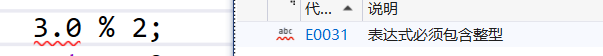移位操作符

<<左移操作符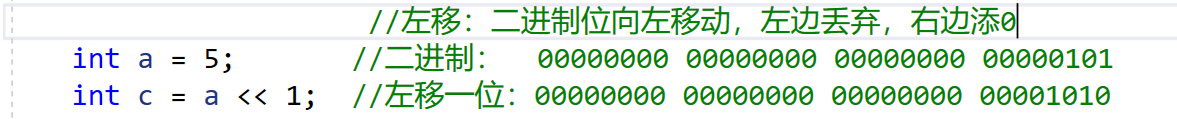>>右移操作符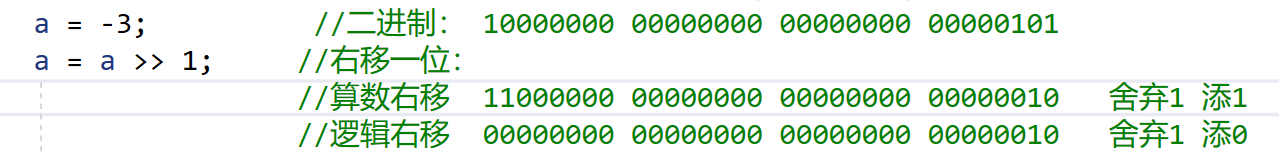a>>-1这样移位错误，C语言未定义。

位操作符

//   00000000 00000000 00000000 00100010
//   00000000 00000000 00000000 11010110
// & 00000000 00000000 00000000 00000010
// | 00000000 00000000 00000000 11110110
// ^ 00000000 00000000 00000000 11110100

&两操作数二进制位都为真（1）结果为真（1）否者为假（0）
|两操作数二进制位为假（0）结果为假（0）否者为真（1）
^一真（1）一假（0）结果为真（1），否者为假（0）
~二进制位按位取反，1变0，0变1

//尝试写一下这个代码
include <stdio.h>
int main()
{
int num1 = 1; //00000000 00000000 00000000 00000001
int num2 = 2; //00000000 00000000 00000000 00000010
num1 & num2; // 00000000 00000000 00000000 00000000
num1 | num2; // 00000000 00000000 00000000 00000011
num1 ^ num2; // 00000000 00000000 00000000 00000011
return 0;
}

//方法一
#include<stdio.h>
int main()
{
int a=3; // 00000000 00000000 00000000 00000011
int b=5; // 00000000 00000000 00000000 00000101
a=a^b;  //  00000000 00000000 00000000 00000110
b=a^b;  //  00000000 00000000 00000000 00000011
a=a^b;   // 00000000 00000000 00000000 00000101
}

^异或操作符的性质
a^a=0;
a^0=a;

//方法二
#include<stdio.h>
int main()
{
int a=3;
int b=5;
a=a+b;  //a=8
b=a-b; // b=3
a=a-b; // a=5
}

//方法一
#include<stdio.h>
int main()
{
int n=10;
int count=0;
while(n)
{
if(n%2==1)
{
count++;
}
n>>=1;
}
printf("输入二进制位1的个数：%d",count);
}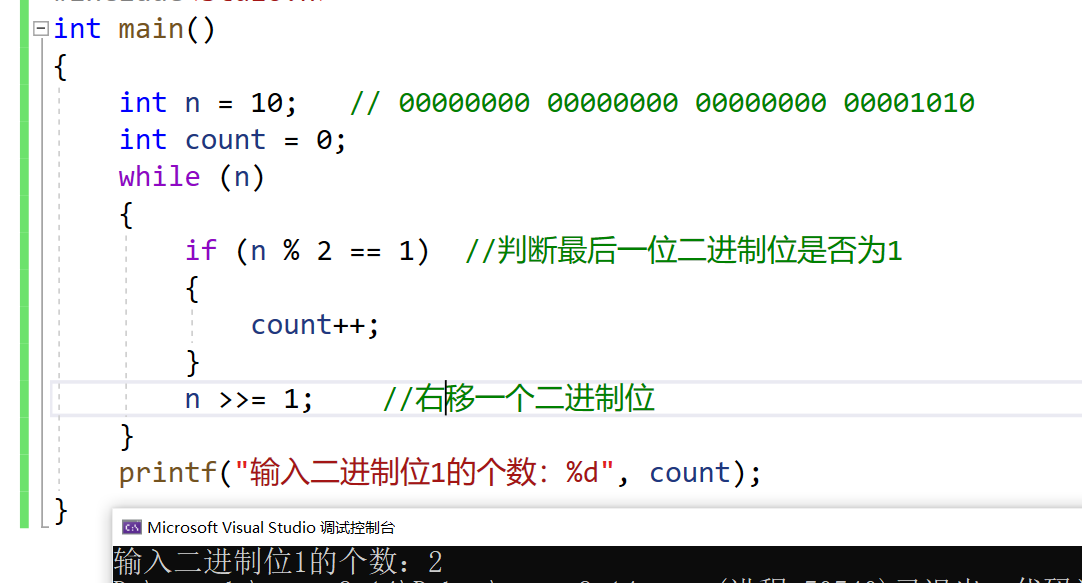思考上面的代码是否存在问题
n为负数时？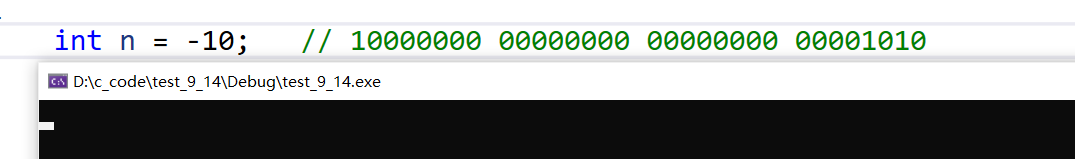可以看到程序将会一直死循环下去。

//方法二
#include<stdio.h>
int main()
{
int i=0;
int count=0;
int num=-3;
for(i=0;i<32;i++)
{
if((num>>i)&1==1)   //移位并且判断最后一位是否为1
count++;
}
return 0;
}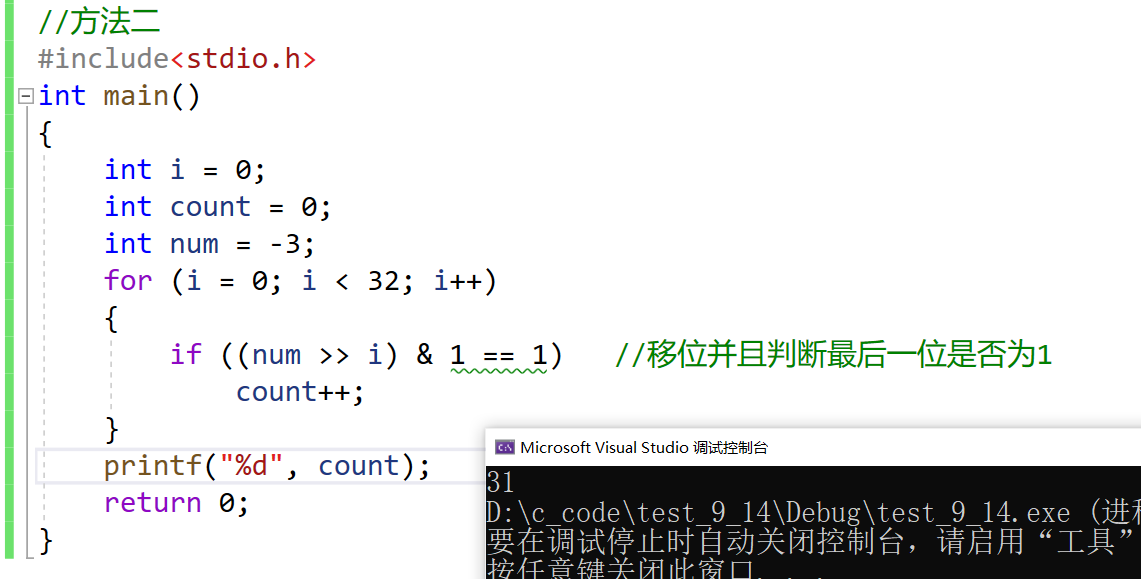//方法三
#include <stdio.h>
int main()
{
int num = -1; // 10000000 00000000 00000000 00000001
//补码  11111111 11111111 11111111 11111111
int i = 0;
int count = 0;//计数
while(num)
{
count++;
num = num&(num-1);//丢弃最后一位1
}
printf("二进制中1的个数 = %d\n",count);
return 0;
}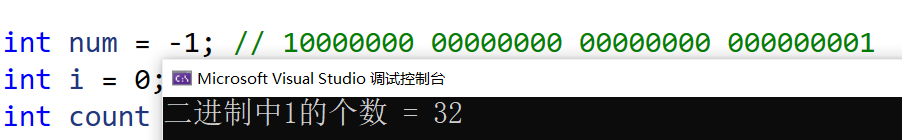上面这个代码是不是很神奇，一般人想不到，这就是代码的魅力！

赋值操作符

#include<stdio.h>
int main()
{
int weight=180;
weight=125;  //不满意可以改变
//连续赋值
int a=13,b=0,c=0;
a=b=c=6;
//连续赋值操作缺点不易调试
}

+= -= *= /= %=

a+=2; ===>   a=a+2;
a*3;  ===>   a=a*3;
//其他运算符一个道理
....

单目操作符

//单目操作符就是只有一个操作数的操作符！
+   -   !   sizeof()   ++  --  ~  *  (类型)

+ -

a=-5;  //-5这里的-指的是单目操作符！
b=+5; //+5 +可以省略！

while(a!=0)   //这里就是！逻辑反操作符
{
count++;   //a不为0count++；
}
while(!a)
{
count++;   //a为0count++；
}

sizeof

sizeof是比较特殊的一个操作符,并不是函数！

int main()
{
int a = -10;
int* p = NULL;
printf("%d\n", !2);
printf("%d\n", !0);
a = -a;
p = &a;
printf("%d\n", sizeof(a));
printf("%d\n", sizeof(int));
printf("%d\n", sizeof a);      //这样写行不行？
printf("%d\n", sizeof int);//这样写行不行？
return 0;
}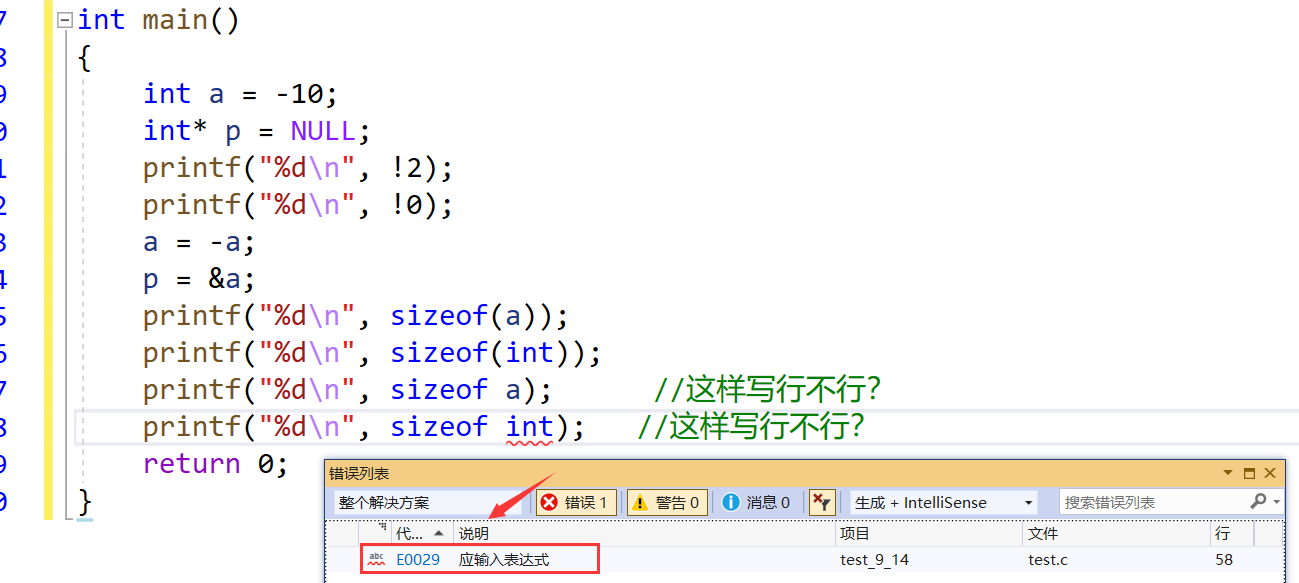sizeof和数组

#include <stdio.h>
void test1(int arr[])
{
printf("%d\n", sizeof(arr));//(2)
}
void test2(char ch[])
{
printf("%d\n", sizeof(ch));//(4)
}
int main()
{
int arr = {0};
char ch = {0};
printf("%d\n", sizeof(arr));//(1)
printf("%d\n", sizeof(ch));//(3)
test1(arr);
test2(ch);
return 0;
}

（1）、（2）两个地方分别输出多少？
（3）、（4）两个地方分别输出多少？

（1）40 （2） 40 （3）10 （4） 10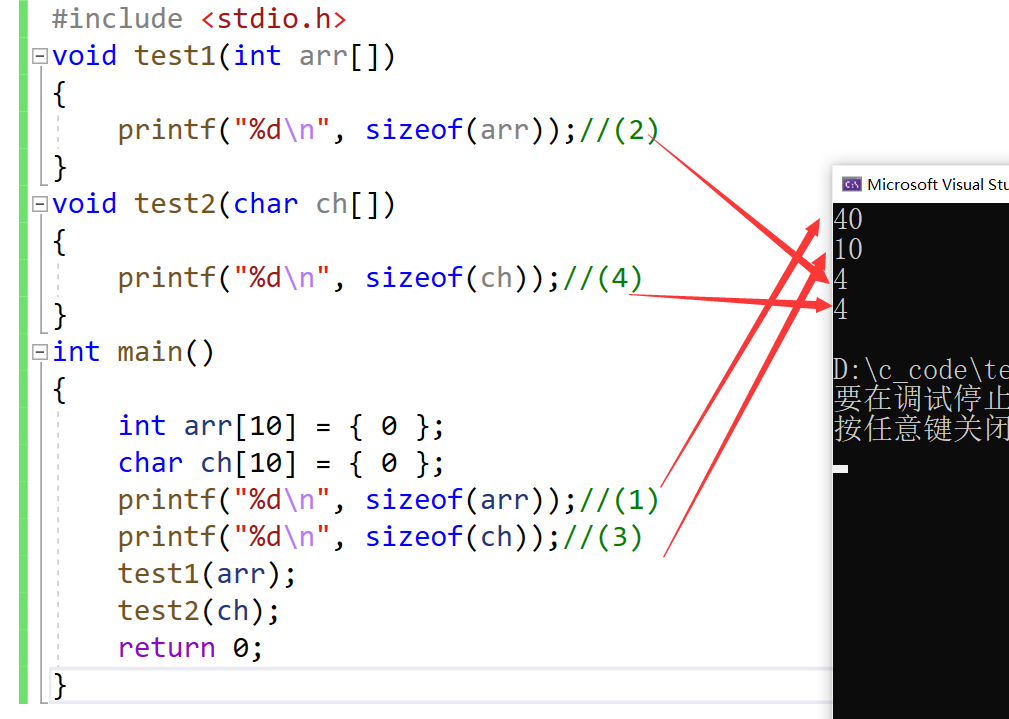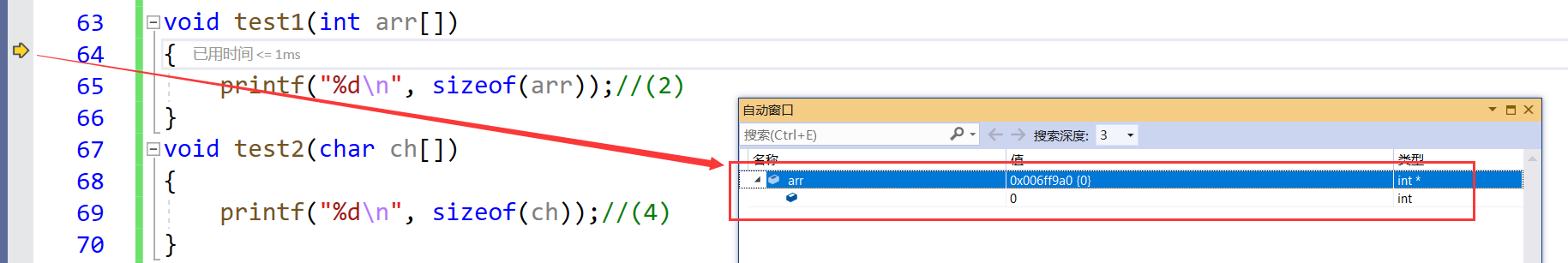++ --

//前置++和--：
#include <stdio.h>
int main()
{
int a = 10;
int x = ++a;
//先对a进行自增，然后对使用a，也就是表达式的值是a自增之后的值。x为11。
int y = --a;
//先对a进行自减，然后对使用a，也就是表达式的值是a自减之后的值。y为10;
return 0;
}

//后置++和--
#include <stdio.h>
int main()
{
int a = 10;
int x = a++;
//先对a先使用，再增加，这样x的值是10；之后a变成11；
int y = a--;
//先对a先使用，再自减，这样y的值是11；之后a变成10；
return 0;
}

关系操作符

> >= < <= == (判断是否等于) ！=（判断不等于）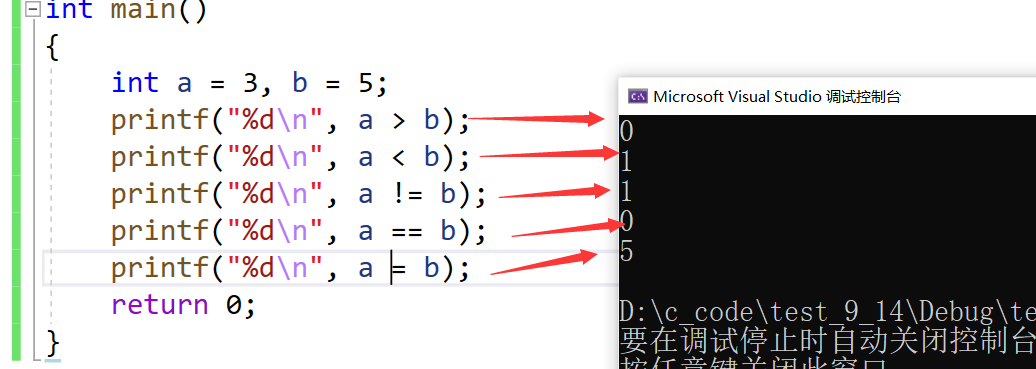逻辑操作符

#include<stdio.h>
int main()
{
int a=3;//00000000 00000000 00000000 00000011
int b=1;//00000000 00000000 00000000 00000001
printf("%d\n",a&b);
printf("%d\n",a|b);
printf("%d\n",a&&b);
printf("%d\n",a||b);

return 0;
}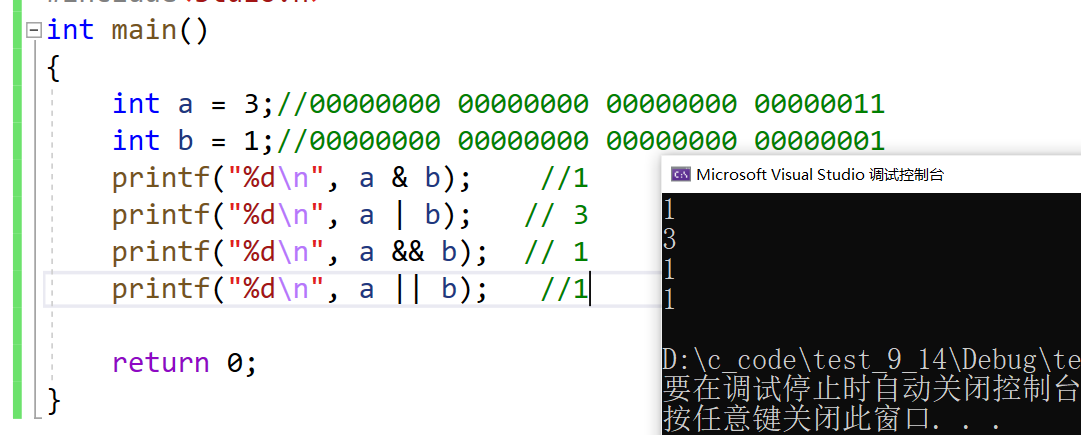&& 只有当两个表达式结果同时为真，结果才为真！
|| 只有当两个表达式结果同时为假，结果才为假！

#include<stdio.h>
int main()
{
int a=3,b=5,c=6,i=0;
i=a++&&++b;
printf("%d %d\n",a,b);
i=a++||++b;
printf("%d %d\n",a,b);
return 0;
}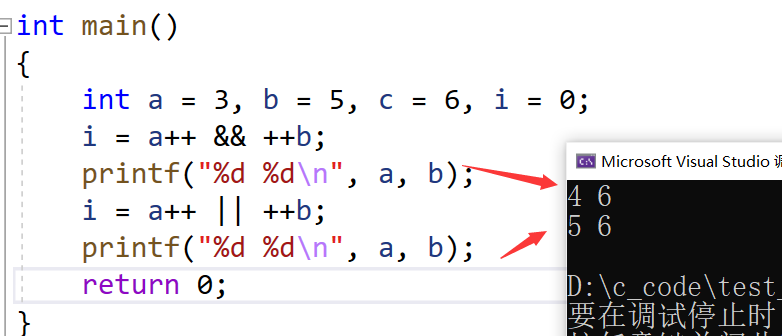我们可以看到，逻辑或||第二个表达式，并没有执行。

条件操作符

exp1 ? exp2 : exp3

#include<stdio.h>
int main()
{
int a=5,b=3,max=0;
//if判断语句求最大值
if(a>b)
{
max=a;
}
else
{
max=b;
}
//条件表达式
a>b?max=a:max=b;
return 0;
}

逗号表达式

exp1，exp2,exp3...expN

#include<stdio.h>
int main()
{
int a=2,b=3,c=5;
int i=(a++,b++,c);
printf("a=%d b=%d c=%d i=%d",a,b,c,i);
return 0;
}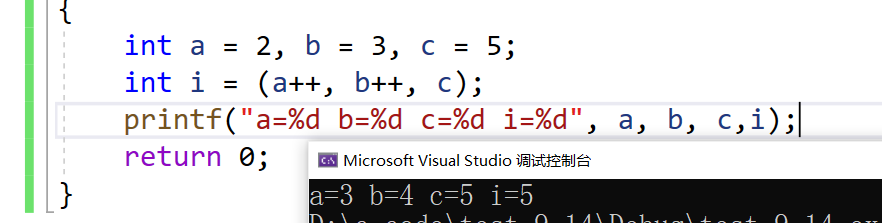其他操作符

[]下标引用操作符 （）函数调用操作符 . ->结构成员访问操作符

[]下标引用操作符

int arr;//创建数组
arr = 10;//实用下标引用操作符。
// [ ]的两个操作数是arr和9。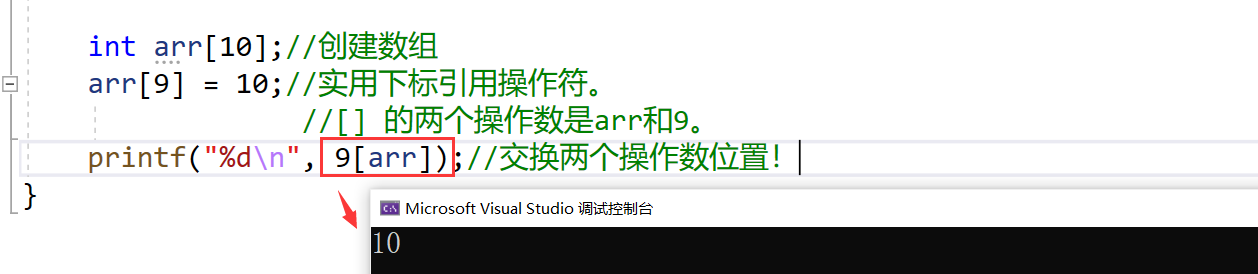()函数调用操作符

( ) 函数调用操作符

#include <stdio.h>
void test1()
{
printf("hehe\n");
}
void test2(const char *str)
{
printf("%s\n", str);
}
int main()
{
test1();//实用（）作为函数调用操作符。
test2("hello bit.");//实用（）作为函数调用操作符。
return 0;
}

. :结构体.成员名
->:结构体指针->成员名

#include <stdio.h>
struct Stu
{
char name;
int age;
char sex;
double score;
}void set_age1(struct Stu stu)
{
stu.age = 18;
}
void set_age2(struct Stu* pStu)
{
pStu->age = 18;//结构成员访问
}
int main()
{
struct Stu stu;
struct Stu* pStu = &stu;//结构成员访问

stu.age = 20;//结构成员访问
set_age1(stu);

pStu->age = 20;//结构成员访问
set_age2(pStu);
return 0;
}